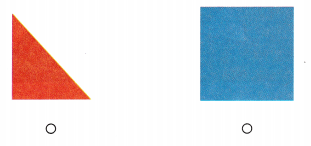# Texas Go Math Kindergarten Lesson 17.2 Answer Key Identify Triangles

Refer to our Texas Go Math Kindergarten Answer Key Pdf to score good marks in the exams. Test yourself by practicing the problems from Texas Go Math Kindergarten Lesson 17.2 Answer Key Identify Triangles.

## Texas Go Math Kindergarten Lesson 17.2 Answer Key Identify Triangles

Essential Question
How can you identify, sort, and describe circles?

ExploreExplanation:
a triangle is a three-sided polygon that consists of three edges and three vertices.
3 sides and 3 corners.

Directions
Use your finger to trace around the triangle. Talk about the number of straight sides and the number of vertices. Draw an arrow pointing to another vertex. Use a pencil or crayon to trace around the triangle.

Share and Show

Question 1.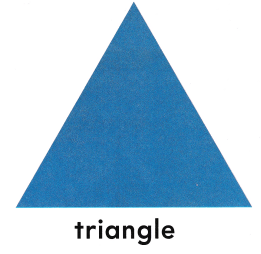A triangle is a three-sided polygon that consists of three edges and three vertices.
3 sides and 3 corners.

Question 2.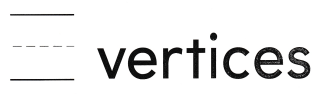Explanation:
A triangle is a three-sided polygon that consists of three edges and three vertices.
3 sides and 3 corners.

Directions
1. Place a counter on each corner, or vertex. Write how many corners, or vertices. 2. Trace around the sides. Write how many sides.

Question 3.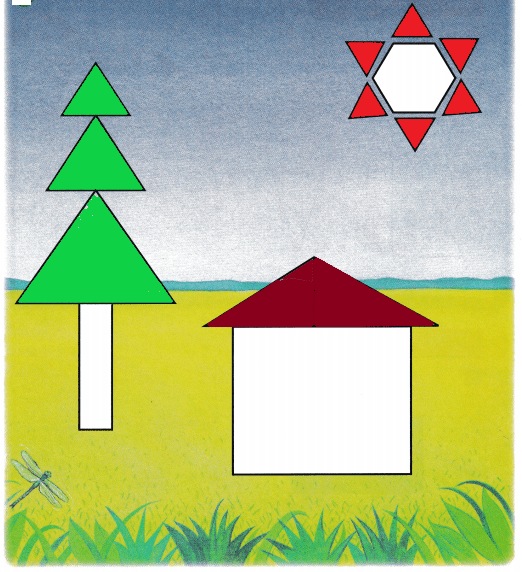Explanation:
Triangles are colored
A triangle has 3 sides and 3 vertices

Directions
3. Color the triangles in the picture.

Home Activity

• Have your child show you an object that is shaped like a triangle.

Problem Solving

Question 4.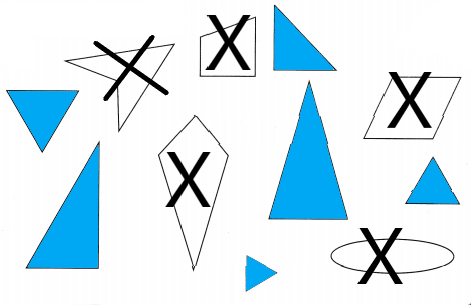Explanation:
Colored the triangles and crossed the shapes that are not triangles
triangles are colored in blue.

Question 5.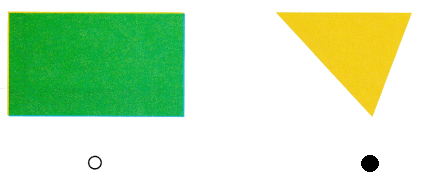Explanation:
A triangle has 3 sides and 3 vertices
The rectangle has 4 sides and 4 vertices
so, marked the second shape.

Directions
4. Sort the shapes. Color the shapes that are triangles. Mark an X on the shapes that are not triangles. 5. Choose the correct answer. Which shape has three sides?

### Texas Go Math Kindergarten Lesson 17.2 Homework and Practice

Question 1.Explanation:
A triangle has 3 sides and 3 vertices
colored all the triangles in red.

Directions
1. Color the triangles in this picture.

Texas Test Prep

Lesson Check

Question 2.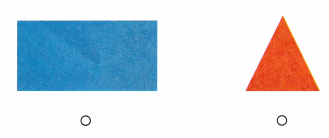Explanation:
A triangle has 3 sides and 3 vertices
the first shape has 4 sides and 4 vertices
so, marked the first shape.

Question 3.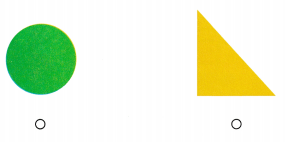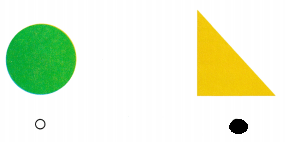Explanation:
A triangle has 3 sides and 3 vertices
so, marked the second shape.

Question 4.